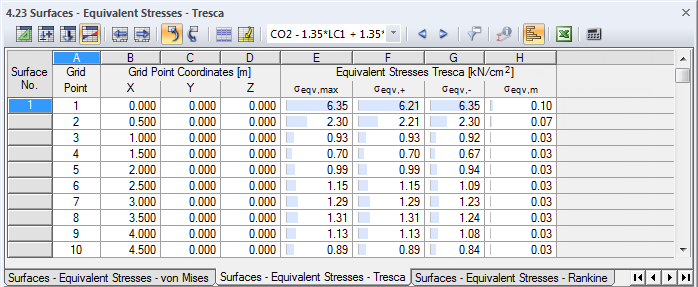# RFEM

Online manuals, introductory examples, tutorials, and other documentation.

# 8.23 Surfaces - Equivalent Stresses - Tresca

To control the graphical display of equivalent stresses of surfaces, select Surfaces → Stresses in the Results navigator (see Figure 8.54). Table 4.23 shows the surfaces' equivalent stresses according to Tresca in numerical form.Image 8.56 Table 4.23 Surfaces - Equivalent Stresses - Tresca

The Grid Point and Grid Point Coordinates table columns correspond to those of the previous result table 4.22 Surfaces - Equivalent Stresses - von Mises.

The approach by Tresca is also known as the "maximum shear stress theory". It is assumed that failure is caused by the maximum shear stress. As this hypothesis is especially applicable for brittle materials, it is frequently used in mechanical engineering.

The equivalent stresses according to Tresca are determined as follows:

 σeqv,max Maximum equivalent stress on the positive or negative side of the surface σeqv,+ Equivalent stress on the positive side of the surface σeqv,− Equivalent stress on the negative side of the surface σeqv,m Membrane equivalent stress